Engineering Entrance Sample Papers

JEE Mains Mathematics Sample Paper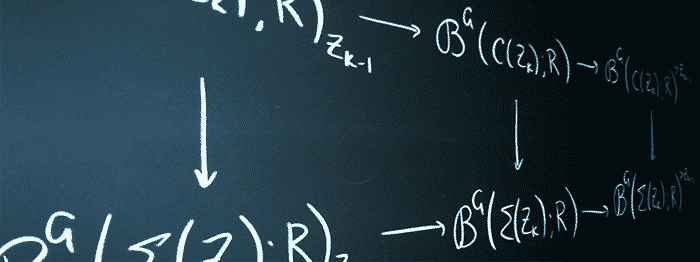This sample paper is based on JEE Mains B Tech Entrance Exam maths exam pattern, for complete details about JEE Exam follow the link: JEE Exam

Question: If the distance of any point (x, y) from origin is defined as d(x, y) = max {|x|, |y|}, then the locus of the point (x, y), where d (x, y) = 1 is
(a) a circle
(b) a square
(c) a triangle
(d) none of these

Question: If the distances of two points P and Q from the focus of a parabola y= 4x are 4 and 9 respectively, then the distance of the point of intersection of tangents at P and Q of the parabola from the focus is
(a) 8
(b) 6
(c) 5
(d) 13

Question: There are 10 seats in a double decker bus, 6 in the lower deck and 4 on the upper deck. Ten passengers board the bus, of them 3 refuse to go to the upper deck and 2 insist on going up. The number of ways all the passengers can be seated is
(a) 60
(b) 10
(c) 100
(d) 50

Question: The area bounded by the curve f(x) = x + sinx and its inverse function between the ordinates x = 0 to x = 2is

(a) 4square units
(b) 8square units
(c) 4 square units
(d) 8 square units

Question: Let P be a point inside triangle ABC and PD, PE and PF be the perpendiculars from P on BC, CA and AB respectively. Then a.PD + b.PE + c.PF
(a) is s, for any choice of P
(b) is 2D for any choice of P
(c) depends on the choice of P
(d) depends on the nature ofABC

Question: The orthocenter of the triangle formed by the points (0, 0), (4, 0) and (3, 4) is
(a) (2, 0)

(b)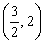(c)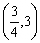(d)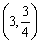Question:

If one of the diameters of the circle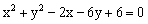is a chord to the circle with centre at (2, 1), then the radius of the circle is

(a)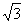(b)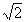(c) 3

(d) 2

Related: BITSAT Maths Sample Paper

Question: A bridge is in the shape of a semi-ellipse. It is 400 meters long and has a maximum height of 10 metres at the middle point. The height of the bridge at a point distant 80 meters from one end is
(a) 4 metres
(b) 2 metres
(c) 8 metres
(d)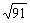metres

Question:

Equation of the straight line making equal angles with the straight lines x + y =1 and
2x + 3y =1 and passing through the point (1,2) may be given as

(a)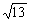(x + y -3) = ±(2x + 3y -8)
(b)(x + y +1) = ±(2x + 3y + 6)
(c)(x + y + 5) = ±(2x + 3y + 9)
(d) none of these

Question: The middle point of the line segment joining A(3, –1) and B(1, 1) is shifted by two units at a certain angle to the line segment AB. If the co–ordinates of the point in the new position is, then the angle is
(a)/2
(b)/6
(c) 0
(d)/4

Question: There are four balls of different colours and four boxes of colours same as those of the balls. The number of ways in which the balls, one in each box, could be placed such that a ball does not go to box of its own colour is
(a) 8
(b) 7
(c) 9
(d) None of these
Ans:- (c)

Question: If A and B are square matrices of order 3 such that | A | = –1, | B | = 3, then | 3AB | =
(a) – 9
(b) – 81
(c) – 27
(d) 81
Ans:- (b)

Related: JEE Entrance Exam

Question: The resultant of two forces P and Q is R. If Q is doubled, R is doubled and if Q is reversed, R is again doubled. If the ratio P2 : Q2 : R2 = 2 : 3 : x, then x is equal to
(a) 5
(b) 4
(c) 3
(d) 2
Ans:- (d)

Question: An engine and train weight 420 tons and the engine exerts a force of 7 tons. If the resistance to motion be 14 lbs. wt. per ton, then the time, the train will take to acquire a velocity of 30 m/hr. from rest is
(a) 2.2 min
(b) 2.6 min
(c) 2.8 min
(d) 3 min
Ans:- (a)

Question: An unbiased coin is tossed. If the result is a head, a pair of unbiased dice is rolled and the number obtained by adding the numbers on the two faces is noted. If the result is a tail, a card from a well shuffled pack of eleven cards numbered 2, 3, 4,…….,12 is picked and the number on the card is noted. The probability that the noted number is either 7 or 8, is
(a) 0.24
(b) 0.244
(c) 0.024
(d) None of these
Ans:- (b)

Question: Two variables x and y are related by the linear equation ax + by + c = 0. The coefficient of correlation between the two is + 1, if
(a) a is positive
(b) b is positive
(c) a and b both are positive
(d) a and b are of opposite sign
Ans:- (d)

Related: JEE Sample Papers

Question: The root of the equation x3 + x – 3 = 0 lies in interval (1, 2) after second iteration by false position method, it will be in
(a) (1.178, 2.00)
(b) (1.25, 1.75)
(c) (1.125, 1.375)
(d) (1.875, 2.00)
Ans:- (a)

Question: Negation of the proposition : If we control population growth, we prosper
(a) If we do not control population growth, we prosper
(b) It we control population growth, we do not prosper
(c) We control population but we do not prosper
(d) We do not control population, but we prosper
Ans:- (c)

Question: (1101101)2 is
(a) 81
(b) 121
(c) 109
(d) 92
Ans:- (c)

Question: The tangents are drawn from the point (4, 5) to the circle x2 + y2 – 4x – 2y – 11 = 0. The area of quadrilateral formed by these tangents and radii, is
(a) 15 sq. units
(b) 75 sq. units
(c) 8 sq. units
(d) 4 sq. units
Ans:- (c)

Question: The equation of the common tangent to the curves y2 = 8x and xy = –1 is
(a) 3y = 9x + 2
(b) y = 2x + 1
(c) 2y = x + 8
(d) y = x + 2
Ans:- (d)

Question: Let the value of p = (x + 4y)a + (2x + y + 1)b and q = (y – 2x + 2)a + (2x – 3y – 1)b, where a and b are non-collinear vectors. If 3p = 2q, then the value of x and y will be
(a) – 1, 2
(b) 2, – 1
(c) 1, 2
(d) 2, 1
Ans:- (b)

Question: The resultant of P and Q is R. If P is reversed, Q remaining the same, the resultant becomes R’. If R is perpendicular to R’, then
(a) 2P = Q
(b) P = Q
(c) P = 2Q
(d) None of these
Ans:- (b)

Question: A parachute weighing 112 lbs. wt. falling with a uniform acceleration from rest, describes 16 ft. in the first 4 sec. Then the resultant pressure of air on the parachute is
(a) 85 lbs. wt.
(b) 95 lbs. wt.
(c) 105 lbs. wt.
(d) 115 lbs. wt.
Ans:- (c)

Question: The coefficient of correlation between x and y is 0.6, then covariance is 16. Standard deviation of x is 4, then the standard deviation of y is
(a) 5
(b) 10
(c) 20/3
(d) None of these
Ans:- (c)

Related: Physics Optics Sample Paper

Question: The value of the root nearest to the 2 after first iteration of the equation x4 – x – 10 = 0 by Newton-Raphson method, is
(a) 2.321
(b) 2.125
(c) 1.983
(d) 1.871
Ans:- (d)

Question: The decimal equivalent of (264)8 is
(a) 180
(b) 170
(c) 166
(d) None of these
Ans:- (a)